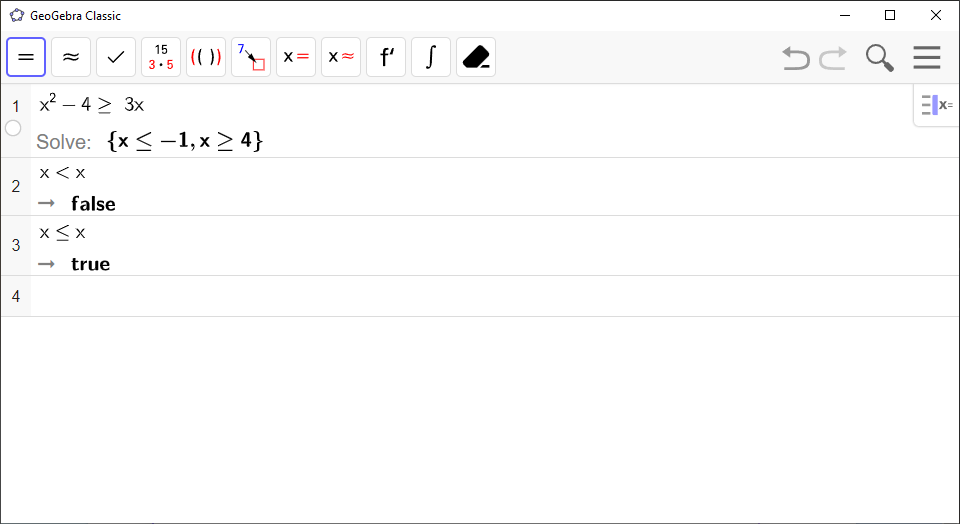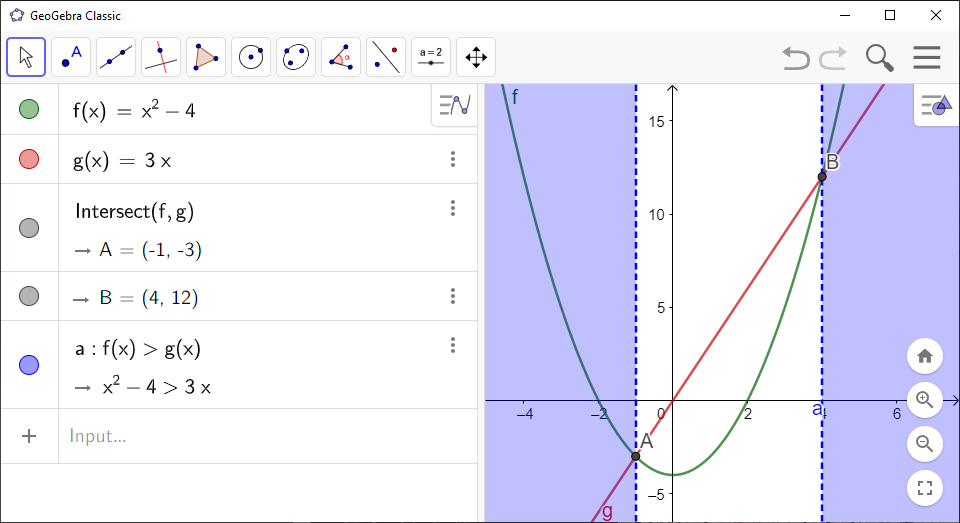# How to Solve Inequalities in GeoGebra

You can use `GeoGebra` to solve systems of inequalities. Type `>=` to enter $\ge$, and `<=` to enter $\le$.

`GeoGebra` Instruction 1

### Solutionin`CAS`

1.
Open `CAS` under`View` in`Menu`.
2.
Enter the inequality and press `Solve`to get an exact solution .

Example 1

The image below shows what it looks like in `GeoGebra` if you solve the inequality ${x}^{2}-4\ge 3x$ by following the instructions above.

Note! If `GeoGebra` returns $\left\{\right\}$, then the inequality has no solution. If `GeoGebra` returns `true`, then the inequality is always true. If `GeoGebra` returns `false`, then the expression is never true for any $x$. If `GeoGebra` returns `?`, you have pressed `Solve Numerically`. `GeoGebra` does not allow this. You must use `Solve`.`GeoGebra` Instruction 2

### Solutionin`Graphics View`

1.
Open `Graphics View` in`View`.
2.
Enter the left-hand side of the inequality in `Algebra View` and press `Enter`.
3.
Enter the right-hand side of the inequality in `Algebra View` and press `Enter`.
4.
Select the `Intersect`tool and click the two graphs to find any possible intersections. You’re going to use the intersection points later.

If you don’t get any intersection points, then your inequality is either always true, or never true. Continue to the next step to find out which one of these is the case for your inequality.

5.
If the inequality contains the symbols $>$ or $\ge$, type `f(x)>g(x)` in `Algebra View`. If the inequality contains the symbols $<$ or $\le$, type `f(x)<g(x)` in `Algebra View`. Then press `Enter`.

The solution is the union of all the intervals of the $x$-axis that are covered in blue.

If you didn’t get any intersection points in Step 4, the `Graphics View` window should be covered entirely in blue if your inequality is always true ($x\in ℝ$), or not covered at all if it’s never true ($x\in \varnothing$).

If you did get intersection points in Step 4, use the $x$-coordinates of the intersection points as the boundaries of the intervals. If the blue area extends indefinitely to the right, use $\infty$ instead, or if it extends indefinitely to the left, use $-\infty$ instead. If the equation uses $\ge$ or $\le$, use brackets, i.e. $\left(\phantom{\rule{-0.17em}{0ex}}\left[\right]\right)$ at the boundary points, otherwise use regular parentheses, $\phantom{\rule{-0.17em}{0ex}}\left(\right)$.The picture shows the area where the graph of $f\left(x\right)={x}^{2}-4$ lies above the graph of $g\left(x\right)=3x$ in the coordinate system. This means that the $y$-value of $f$ is greater than the $y$-value of $g$ in the shaded area. From the image you can see that $f\left(x\right)>g\left(x\right)$ when $x\in \phantom{\rule{-0.17em}{0ex}}\left(-\infty ,-1\right)\cup \phantom{\rule{-0.17em}{0ex}}\left(4,\infty \right)$.